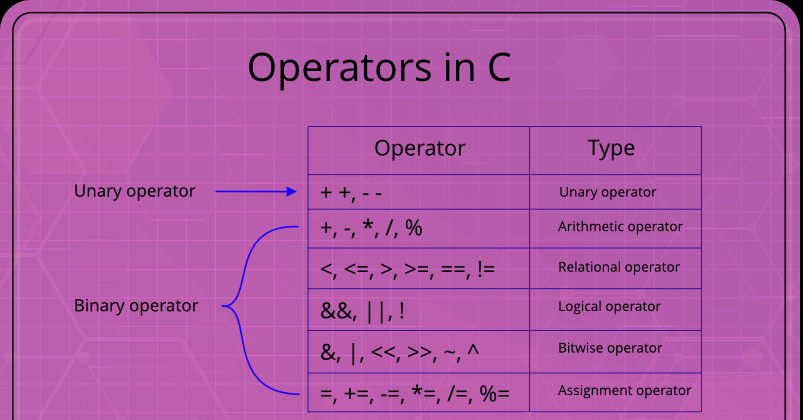## CodeNewbie Community 🌱# Operators in C Programming

In this tutorial, we will learn Operators in c programming, and the types of unary and binary operators, and the difference between the unary operator and binary operator. So let us start.

## What are operators in c?

An operator is a symbol or sign that operates on a value or a variable. In any of the programming languages, there are different-different types of mathematical & logical calculations. To perform the mathematical and logical operations some special symbols are used. For performing Different-different types of calculations the computer needs some symbols which are called an operator. For defining the data identifiers are used.

## What are the types of operators in c?

There are two types of operators. The unary operator and the binary operator.

## Unary operator

The operators which need only one operand for performing the operation are known as Unary operator. In the C language, the unary operators that are supported are of different - different types. For Example: -

Symbols Name Symbols Name
& Address operator * Indirection operator
+ Unary + - Unary -
~ bitwise operator ++ Unary increment operator
: Logical operator -- Unary decrement operator

## Binary operator

Those operators who need two operands for performing the operations are known as binary operators. For example:

### Arithmetic operator

An arithmetic operation will perform mathematical operations like addition, subtraction, multiplication, division, etc.

### Relational operator

This operator checks the relationship between the two operands. If the relation is true then it returns the value 1 and if the relation is false then it returns the value 0.

### logical operator

In the logical operator, the value 1 or 0 is returned depending upon whether the expression result is true or false.

### The ternary and conditional operator

The ternary operator uses three operands instead of one or two. The condition operator checks the condition and assigns the value according to the condition.

### Increment and decrement operator

These operators are used for incrementing or decrementing the value in a variable by 1.

### bitwise operator

During the mathematical operations like addition, subtraction, etc. are converted into a bit-level for making the processing faster.

### Assignment operator

This operator is used to assign a value to the variable. The most common assignment operator is =

### comma operators

It is used to link related expressions together.

### Sizeof operator

By using this operator we can get the size of the data

## What’s the difference between unary operators and binary operators?

Unary operator Binary operator
Performs the action with a single operand Performs the action with two operands
These contain the arithmetic operators These contain arithmetic, logical, conditional, relational operators